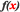# Functions

Nintex for Office 365 forms have the two types of functions available, depending on context.

• Inline functions
• Runtime functions

## Inline functions

Use inline functions to perform additional processing on a text or value, calculated at the time the form is loaded.

For example, you can use inline functions to show or hide a control (toggle the appearance--visible or enabled) in the following situations.

To show or hide a control in this situation Use this expression
The form is in new or edit mode fn-Or(Is New Mode, Is Edit Mode)
The form is in edit mode and the user is a member of the Finance group (in SharePoint) fn-And(fn-IsMemberOfGroup(Finance), Is Edit Mode)

Several functions are provided. Functions can also be used as arguments for other functions.

At the time that the form is loaded, the parsing engine replaces any inserted reference tokens first, and then the resulting text is evaluated for functions. If a function contains another function as an argument, the inner-most function is evaluated first. As reference tokens are replaced first, the reference token can also contain function syntax for evaluation.

Note: Functions cannot refer to named controls.

## Runtime functions

Use runtime functions to perform calculations for a set of values. You can add runtime functions to conditions for rules.

Following are some example runtime functions.

Example runtime function Description
intValue1+intValue2 Adds the named controls intValue1 and intValue2.
sum(decAmount) Totals all values for instances of the named control decAmount within a Repeating Section control.
1+txtValue1 Concatenates the number 1 and the string value from the named control txtValue1.
1+"-"+txtValue1 Concatenates the number 1, a hypen, and the string value from the named control txtValue1.

To add a runtime function to a rule condition

1. In the Rules pane, select the rule that you want to edit.
2. Clickto the right of Condition.

The Formula Builder dialog box appears.

3. In the Formula text box, place the cursor at the location at which you would like to insert the runtime function (or highlight the text that you want to replace with the inserted runtime function).
4. In the Insert Reference pane on the right of the Formula Builder dialog box, expand the Runtime Functions section.
5. Double-click the runtime function that you want to insert.

The function template for that function is inserted.

For example, the function template sum([set of values]) appears for the function sum.

6. In the Formula text box, edit the function template as needed to reference named controls or other references.

For example, to edit the function template sum([set of values]), remove the text "[set of values]" and then expand the Named Controls section of the Insert Reference pane to insert each named control that you want to reference in the sum function.

Also see:

Forms